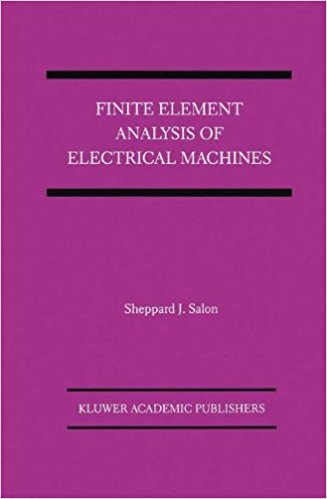# Finite Element Analysis of Electrical MachinesAuthor:
Sheppard J. Salon
Publisher:
Springer 1995
Published in 1995, this was the first book devoted solely to the finite element analysis of electrical machines.

This book covers the two-dimensional finite element analysis of electrical machines from its basics all the way to its practical application in synchronous and induction machines. Illustrated with many results and several tutorial-like examples, it explains how to setup and solve a finite element simulation. It then discusses how to compute losses, resistances, inductances, force and torque from the resulting magnetic potential. This book has helped me with many practical issues, especially:

• How to solve non-linear electromagnetic problems with the Newton-Raphson iterative method.
• How to use cubic splines for the interpolation and extrapolation of the B-H curve.
• How to correctly model a stack of laminations.

Since this book was published back in 1995, more recent topics such as permanent magnet machines or iron loss calculations are not at all or only very elementarily treated. Anyway, if you can put your hands on an official Indian reprint of this book, it is definitively worth the money.